Constructive Solid Geometry Question submitted by (13 November 2000)Return to The Archives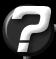I have a question which I hope you will be able to answer for me (or at least point me in the right direction). It's to do with Constructive Solid Geometry. Im currently writing an editor for my engine which is very similar in most respects to worldcraft. Now what I need to do is to have the ability to CARVE one object from another, for example, if I have a solid cube, and i place a cylinder partially inside that cube, and hit CARVE, it will subtract the shape of that cylinder from the cube. This will be entirely in the editor, so i need to know how to split my geometry/faces up to subtract (and maybe even add at a later date) brushes from/to other geometry. The only example I have ever seen which touches on this subject, uses BSP trees to do a lot of the work, but I'm not using BSP trees at all.Hoping you can shed any light on this huge void of information =)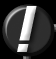What you're looking for is traditionally referred to as Regularized Boolean Set Operations. Originally, these operations were created for use in CSG operations where objects were defined mathematically, not discreetly (i.e. polygonal objects or, "boundary representations".) The answer is somewhat long, so I've broken it up into sections. Regularized Boolean Set Operations: What are they? There are three primary Boolean Set Operations: Subtraction, Union and Intersection: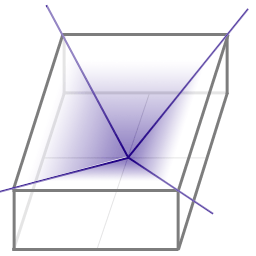Figure 1: Example of the Regularized Boolean Set OperationsSubtraction simply removes the volume of one object from the volume of another object. Union joins the two objects and "removes the stuffing", while intersection results in just the volume that is occupied by both objects.Note that these operations can be written using a formula such as "A op B" where A is the sphere, and B is the box. In the case of subtraction, op is the subtraction symbol, resulting in an operation of "A – B" (the volume of the box is subtracted from the volume of the sphere.)References The two most popular methods for Regularized Boolean Set Operations are (1) the BSP approach you mentioned, based on the paper "Set Operations on Polyhedra Using Binary Space Partitioning Trees" by Thibault & Naylor and (2) a technique based on the paper "Constructive Solid Geometry for Polyhedral Objects" (Laidlaw, Trumbore & Hughes) which was published in the '86 SIGGRAPH.If you happen to have a copy of "Computer Graphics Principles and Practice" handy, you can look in section 12.6.4 which glazes over the BSP approach, or peek at section 12.5.3 for a brief reference on the second technique.The Laidlaw approach I've written code to do this once before and chose the Laidlaw approach because I wasn't using BSP trees with my work. Looking back, I'm not convinced that I made the right decision. You should investigate them both and choose for yourself. In the meantime, I'll explain what I know about the Laidlaw approach, which does work very well.Closed Objects When calculating the result of a Boolean Operation, it's important that both input objects (we'll call them A and B) are completely closed. By this, I mean that each object must define a closed surface with shared (or "merged") vertices. If your object contains a t-junction, your object is not technically closed.One way to determine if an object is closed, is to visit each polygon and make sure that each edge of that polygon shares an edge with another polygon. If you find an edge that's not shared, then the object is not closed. A shared edge is defined as two vertices from one polygon are shared with two vertices from another polygon, with opposite winding order between the two edges: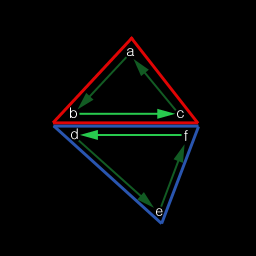Figure 2: Opposite winding order for shared edges Splitting Up The Objects In order to perform any Boolean Operations, we need to bisect the polygons of each object that intersect polygons of the other object. To clarify, this means that if a polygon from object A intersects a polygon from object B, then both polygons need to be bisected by one another: object A's polygon is bisected by the plane of object B's polygon, and object B's polygon is bisected by the plane of object A's polygon. The trick is, you only need to do this when there is an actual intersection of the two polygons. There are a few ways of going about doing this, but I'll describe a simple technique.Start by making two copies of each object; we'll call these objects A' and B'. We'll need to do this because we'll be replacing polygons with the multiple pieces from any bisection that takes place.Visit each polygon in A' and compare its bounding box with the bounding box from each polygon in B'. If no overlap exists, then there's no intersection (and no bisection is required.) If there is an overlap, go ahead and attempt to bisect each polygon by the other polygon's plane. If both polygons end up being bisected, then replace the original polygons from both objects with their bisected counterparts. However, if one polygon or the other ends up not being bisected, then leave both polygons unmodified.A' and B' are now 'refined' for the Boolean Operations. The only thing left to do is to figure out which polygons from each object is part of the result.Quick Recap At this point, we have two closed objects that probably share some volume between the two (i.e. they're embedded.) At every point where the surfaces of the two objects penetrate each other, we've sliced the surfaces of the objects.What we need to do now is to remove the polygons that don't belong. Some polygons are removed because they are interior polygons (i.e. polygons from one object within the volume of the other object) and some polygons are removed because they are exterior polygons. Depending on the Boolean Operation being performed, one group of polygons or the other from each object will need to be removed. For example, if we're trying to subtract object B from object A, part of the task is to remove the polygons of object A that are within the volume of object B.If you want your Boolean operations to be robust, you'll also need to detect and deal with shared polygons. Shared polygons are those polygons that overlap, as in figure 3: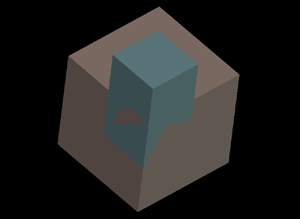Figure 3: A situation where two embedded objects have polygons that share surface area. Note that because these polygons lie on the same plane and overlap, we see artifacts in the z- buffer. Also note that these polygons only partially overlap. Fortunately, after we slice up our objects, we'll end up with polygons that are completely overlapped, or not overlapped at all. Because of this, these polygons are easy to detect (the normals and the D values of both polygons are all within an epsilon distance.)Removing The Unnecessary Polygons The basic premise of this next step is to remove polygons from our refined objects (A' and B') that correspond to the result. The result, in this case, depends on the Boolean Operation being performed.Here are the necessary operations: Subtraction Remove polygons from A' that are within the volume of B (remove shared). Remove polygons from B' that are outside the volume of A (remove shared). Invert the normals of the remaining polygons from B'. Merge A' and B' to get the result. Union Remove polygons from A' that are within the volume of B (remove shared). Remove polygons from B' that are within the volume of A (keep shared). Merge A' and B' to get the result. Intersection Remove polygons from A' that are outside the volume of B (remove shared). Remove polygons from B' that are outside the volume of A (keep shared). Merge A' and B' to get the result. Note 1: We are removing polygons from the refined objects A' or B' that are within/outside the original objects (A or B). This is not a misprint. Doing this increases our accuracy and reduces the amount of work required.Note 2: In some cases we keep the shared polygons. This is necessary because the shared polygons from the other object are kept, and we can't simply delete both polygons (except in the case of subtraction.) Before we can consider our overview of Boolean Operations complete, we need to cover one last topic... Determining If A Polygon Is Interior, Exterior Or Shared To determine if a polygon is within the volume of an object, we'll need a ray that points outward away from the polygon, along it's normal. So we start by creating a ray that has its direction set to the polygon's normal and originates at the center of the polygon. We then locate all of the polygons from the test object to see which ones the ray hits. Note that we're only interested in those intersections that actually intersect the test object's polygons. This usually means that we need to perform a ray/plane intersection, and then determine if that point is within the boundaries of the test object's polygon.Our goal is to locate the shortest intersection distance (usually referred to as 'time') that is positive. Negative distances can be ignored.Note that if time is within an epsilon to zero (i.e. |time| < epsilon) then the polygon is considered shared.Once we know which polygon (from the test object) had the nearest intersection, we need to know if that polygon is facing our input polygon or not. If it is, (i.e. a dot product of the two normals is positive) then the input polygon is inside the test object. If the dot product is negative, then the input polygon is outside the test object.It is quite possible that we might not find any intersection at all. This simply means that our polygon is outside the test object.Note that on occasion, a dot product will be near zero (|dot| < epsilon). In these cases, it will be unclear as to weather the polygon is an interior or exterior polygon. A solution to this is to randomly perturb the normal of the ray slightly and try again. Because of this, it's wise to use the normal of the input polygon in the dot product calculation, rather than the normal of the ray, since the ray may have been perturbed.This may seem like a rather extensive way to determine if a polygon is inside or outside of an object, but it is necessary because this allows us to perform Boolean Operations on non-convex objects.Summary Rather than typing a lot, I'm just going to add some more images, to help visualize what's going on...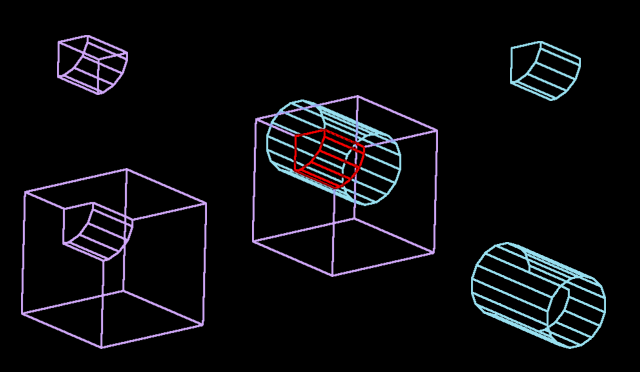Figure 4: Composite of a subtraction operation Figure 4 shows a composite of an entire Boolean Operation after bisections. In the upper-left corner, we have the interior (inside the cylinder) polygons of the box. The lower left corner shows the exterior of the box. The upper-right corner shows the interior (inside the box) of the cylinder and the lower-right corner shows the exterior of the cylinder. The center of the image shows each of the four pieces overlaid in their original positions with the interiors highlighted in red.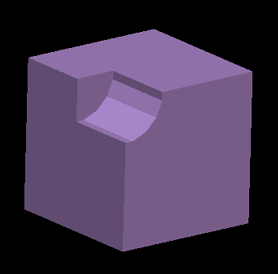Figure 5: The result of a subtraction operation using the pieces from figure 4. Helpful Features If you were implementing this for an editor, I would recommend adding a couple other painless features. First, add a "split" operation. A split performs the polygon bisections of an object by another object, but does nothing else. This offers your artists a very unique way to tessellate an object for easy manipulation.Also, allow the Boolean Operation to keep either of the two input objects. If you think of the operation in terms of: "sphere – box = result" then the Boolean Operation usually calculates the result, and deletes the original objects. However, when doing many Boolean Operations, it might be wise to keep one or the other (or both) of the input objects.In Closing Writing code to perform Regularized Boolean Set Operations is not trivial. Making it robust is tricky to say the least. And because of the need for epsilons, you may find that you will never get it exactly to your liking. However, know that even the advanced modeling packages today can result in disappearing objects as a result from accuracy loss during Boolean Operations.Response provided by Paul Nettle